Courses

# CAT Quantitative Aptitude MCQ - 1

## 34 Questions MCQ Test CAT Mock Test Series 2020 | CAT Quantitative Aptitude MCQ - 1

Description
This mock test of CAT Quantitative Aptitude MCQ - 1 for CAT helps you for every CAT entrance exam. This contains 34 Multiple Choice Questions for CAT CAT Quantitative Aptitude MCQ - 1 (mcq) to study with solutions a complete question bank. The solved questions answers in this CAT Quantitative Aptitude MCQ - 1 quiz give you a good mix of easy questions and tough questions. CAT students definitely take this CAT Quantitative Aptitude MCQ - 1 exercise for a better result in the exam. You can find other CAT Quantitative Aptitude MCQ - 1 extra questions, long questions & short questions for CAT on EduRev as well by searching above.
QUESTION: 1

### If the sum of the roots of the quadratic equations mx2 + (2m - 1)x + 4 and (3m + 1)x2 - 6x + (2m - 3) are equal, then find the sum of values of m.

Solution:

The sum of the roots of a quadratic equation ax2 + bx + c is -b / a

-(2m - 1) / m = 6 / (3m + 1) - 6m2 + m + 1 = 6m - 6m2 - 5m + 1 = 0 m = -1 or 1/6 Thus, the sum of the values of m = -(5/6) Hence, option 3.

QUESTION: 2

### If the last day of the year 1899 is second to the last day of the week, then find the day of the week on which the date 21st April, 1904 falls. Assume that a week starts on Sunday.

Solution:

The number of days between 31st December 1899 and 31st December 1903 = 365 x 4 = 1460
The number of days between 31st December 1903 and 21st April 1904 = 31 + 29 + 31+21 = 112
Total number of days = 1572 Dividing 1572 by 7 the remainder = 4

Hence, 21st April 1904 falls on Tuesday.
Hence, option 2.
Alternatively,
We know that in a non-leap year there are 52 weeks and 1 odd day.
There is no leap year between 1899 to 1903. Hence, there are 4 odd days.
Number of odd days between 31st December 1903 and 21st April 1904 = 3 + 1 + 3 + 0 = 7
Thus, there are total 4 odd days between the given time frame. 21st April 1904 will be a Tuesday.Hence, 21st April 1904 falls on Tuesday.
Hence, option 2.
Alternatively,
We know that in a non-leap year there are 52 weeks and 1 odd day.
There is no leap year between 1899 to 1903. Hence, there are 4 odd days.
Number of odd days between 31st December 1903 and 21st A pril 1904 = 3 + 1 + 3 + 0 = 7
Thus, there are total 4 odd days between the given time frame. 21st April 1904 will be a Tuesday.

Hence, option 2.

QUESTION: 3

### What will be the maximum volume that can be obtained by rotating a right an gled triangle of dimensions 6, 8 and 10 units.

Solution:

The maximum volume will be obtained when it is rotated about the smaller side rather than the hypotenuse.
Radius = 8 units and the height = 6 units.
Thus, the volume = (1 / 3) x (π x 64 x 6) = 128π units.
Hence, option 4.

*Answer can only contain numeric values
QUESTION: 4

The price of 1 pencil is subtracted from the price of 5 pens is equal to one fifth of the price of 3 pencils and 7 pens. Find the price of pencil as a percentage of the price of pen.

Solution:

Let the price of one pencil be x and the price of one pen be y.
Now, (5y - x) = (3x + 7y)/5

⇒ 25y - 5x = 3x + 7y

⇒ x = 2.25y
Thus, the price of pencil as percentage of the price of pen = (2.25y /y) x 100

= 225%

QUESTION: 5

How many subsets of S = (1, 2, 3 , . . . , 400) have the product of their elements an even number?

Solution:

For a given set containing N elements, the number of non-zero subsets = 2N - 1

Number of non-zero subsets in S = 2400 - 1

The product of the elements of a subset will be odd when all the elements of the subset are odd

The odd elements in S are Sodd = (1, 3, 5 , ..., 399) Number of non-zero subsets in Sodd = 2200-1

Number of subsets where product of its elements is even = (2400 - 1) -(2200-1) = 2400 - 2200

Hence, option 1.

QUESTION: 6

Find the maximum area of the isosceles trapezium (in units2) whose unequal sides are 4 units and 6 units. Given that it is inscribed in a circle whose radius is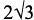Solution:

The maximum area of trapezium will be obtained when the two sides are on the opposite sides of the diameter.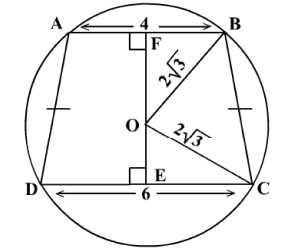The height of the trapezium = OE + OF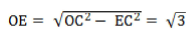And,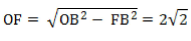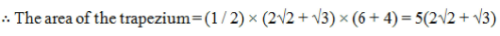Hence, option 2.

QUESTION: 7

The year on year increase in the price of a product can be represented as the sum of two different constants A and B which has variable co-efficients. If the price of the product in 2004 was Rs. 80 and the coeffiicients of A and B for the session 2004-05 are 2 and 5 respectively and for the session 2005-06 are 4 and 1 respectively, then find the relation between A and B if the price increases at a constant rate of 20% per year.

Solution:

The increase in price in 2004-05 session = 80 x 0.2 = Rs.16.
2A + 5B = 16... (i) The increase in price in 2005-06 session = 96 x 0.2 = Rs.19.2 4A + B = 19.2 . . . (i) Solving (i) and (ii), A = 3.125B
Hence, option 3

QUESTION: 8

The average speed of Mumbai metro train which travels from Ghatkopar to Versova is 30 km/hr and the distance between the two stations is 32 km. Which function represents the distance 'd(t)' remaining in a trip from Ghatkopar to Versova after a certain time 't' from the start?

Solution:

We know that,

Distance remaining = Total distance - Distance travelled

d(t) = 32 - 30t

QUESTION: 9

In a bag there are 3 blue coloured balls, 6 green coloured balls, 2 white coloured balls and 5 yellow coloured balls. If 2 balls are selected at random from the bag, then what is the probability that none of them is blue? (Enter the correct option number)

Solution:

Other than blue any other can be selected.
Hence, 2 out of 13 can be selected in 13C2 ways and total ways of selecting 2 balls out of 16 is 16C2 ways.
The required probability = 13C2/16C2 = 13/20 = 78/120

*Answer can only contain numeric values
QUESTION: 10

Find the value of M in the figure given below,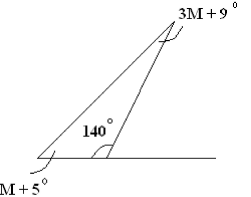Solution:

3M + 9 + M + 5 + 140= 180

4M = 26
M = 6.5°

QUESTION: 11

What is the area of an obtuse angled triangle having two sides as 18 m and 24 m and the angle between them as 150°?

Solution:

In a triangle ABC, if AB, AC and the included angle A are known,

Area = (1/2) xAB x AC x sin A

= (1/2) x 18 x 24 x sin 150

= 216x0.5

= 108 sq.m

Hence, option 2.

*Answer can only contain numeric values
QUESTION: 12

A finance company keeps revising the interest rate it offers every year with a constant percentage increase in rate of interest over the previous year. However the rate of interest remains same for a certain investment over the years of investment. If an investment of one lakh rupees in 2010 at 6% rate of simple interest and an investment of one lakh rupees in 2011 amounts to the same sum in 2014, then find the rate of simple interest offered in 2015.

Solution:

The amount obtained in 2014 by investing 100000 in 2010 = 100000 x (1 + 0.24) = 124000
Let the rate of interest offered in 2011 be r.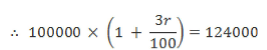r = 8%
The increase percentage in the offered rate = ( 2 / 6 ) x 100 = 33 .3 3 %

Therate of interest offered in 2015 = 8x(1+1/3)4 = 25 .28 %

*Answer can only contain numeric values
QUESTION: 13

6 equally spaced girls are standing along the circumference of a circle of radius 10 m and facing the center. What is the shortest distance between any two girls facing each other?

Solution:

Shortest distance between any 2 girls facing each other will be the diameter of the circle = 10 x 2 = 20 m

*Answer can only contain numeric values
QUESTION: 14

What is the sum of the two middle terms of the arithmetic progression {-17, - 1 3 , . . . , 19}?

Solution:

Since the sum of two middle term s is asked, the series has an even number of terms.
Now for even number of terms we know that, Sum o f the m iddle tw o term of an A P will be equal to the sum of the first and last terms o f the AP = - 1 7 + 1 9 = 2

Alternatively,

First term = a = - 17

Commond difference = d = — 13 — (— 17) = 4
Last term = 19 = — 17 + (n — 1)4

- n = 10
Middle term will be 5th and 6th terms.
Their sum will be = a + 4d + a + 5d = 2a + 9d = 2(— 17) + 9(4) = 2

*Answer can only contain numeric values
QUESTION: 15

In how many ways can you arrange 3 girls and 4 boys in a row such that no boys sit next to each other?

Solution:

3 girls can be arranged in 3! Ways.
Now there are 4 gaps remaining where 4 boys can be arranged in 4! ways.

- Total number of ways = 3 ! x 4 ! = 6 x 2 4 = 144

QUESTION: 16

Of the three numbers, second is twice the first and is also thrice the third. If the average of the three numbers is 77, find the largest number.

Solution:

Let the numbers be x, y and z.

y = 2x and y = 3 z

As the average = 77,

x + y + z = 231

Now,

y/2 + y + yl 3 = 231

y = 126

Hence, option 2.

QUESTION: 17

Establish relation between x and y if

1. x2 + 13x + 40 = 0

2. y2 + 8y + 15 = 0

Solution:

Solving the quadratic equations we get, x = -8 or -5 andy = -5 or -3.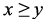Hence, option 4.

QUESTION: 18

A shopkeeper gives a discount of 10 % on the marked price and sells it for Rs. 540 to earn a profit of Rs. 90. Find the profit percentage if the product is marked Rs. 200 above its cost price for the same absolute value of discount.

Solution:

Let the marked price be m.

The selling price = 0.9m

0.9m = 540 m = 600

The discount = Rs. 60

The cost price = Rs. 450

Now, Marked price = Rs. 650

The selling price = 650 - 60 = Rs. 590

The profit = 590 - 450 = Rs. 140

The profit percentage = (140 / 450) x 100 = 31.11%

QUESTION: 19

A thief is running on a circular track of radius 5 m at 1.5 m / s . A policeman whose speed is twice that of the thief arrived at the starting point of the track 4 seconds after the thief. The policeman can either run along the circular track in the direction of the thief or go to the centre and then go to any point on the circle from the centre. Find the minimum distance covered by the thief after the policeman starts chasing him.

Solution:

When the policeman arrives, the thief has traveled 6 meters.
So, the time taken by the policeman to catch the thief along the circular track = 6/1.5 = 4 seconds.
The total distance covered by the policeman =12 meters.
Thus, the minimum distance is when the policeman takes the route via the center i.e. he travels a length of 2 x 5 = 10 meters.

The minimum time taken = 10/3 seconds.

The minimum distance covered by the thief = 1.5 x (10/3) = 5 meters Hence, option 3.

QUESTION: 20

A dishonest shopkeeper mixes pure sugar with some sugar like substance to gain extra profit. The cost price of sugar like substance is 2/3 times the pure sugar. If the shopkeeper wants to gain a profit of 30 % on selling the mixture at the cost price of pure sugar, then find the ratio of sugar like substance to pure sugar in the mixture.

Solution:

Let the cost price of 1 kg of pure sugar be Rs. x.
The cost price of 1 kg sugar like substance = Rs. 2x / 3 The selling price of one kg of mixture = Rs. x.
Let the amount of pure sugar in 1 kg of mixture be y.

The amount of sugar like substanace = (1 —y)

The cost price of mixture = xy + (2x / 3) x (1 - y) = (x / 3) x (2 + y)

x / [(x / 3) x (2 + y)] = 1.3
y = 4/13
Thus, the ratio of sugar like substance to pure sugar = 1 - y : y = 9 : 4

Hence, option 3.

*Answer can only contain numeric values
QUESTION: 21

If x = 44 x 56 x 65 x 710 x 88, then how many factors of x are of the form 6 A2?

Solution:

x = 44 x 56 x 65 x 710 x 88

x = 237 x 35 x 56 x 710

x = (2 x 3) (236 x 34 x 56 x 710)
Now,
Power o f 2 can take values o f 0, 2 , 4 , . . . , 36 = 19

Power of 3 can take values of 0, 2,4 = 3 Power of 5 can take values of 0, 2,4, 6 = 4

Power of 7 can take values of 0, 2,4, 6, 8, 10 = 6

Total number o f factors = 1 9 x 3 x 4 x 6 = 1368 Answer: 1368

*Answer can only contain numeric values
QUESTION: 22

In an examination, 65% of the candidates passed in Mathematics, 55% passed in History, 22% failed in both the subjects and 48909 passed in both the subjects. Find the total number of candidates.

Solution:

Let the percentage total number of candidates who passed in both the subjects be x.
Let the sets M and H represent students who passed in Mathematics and History respectively.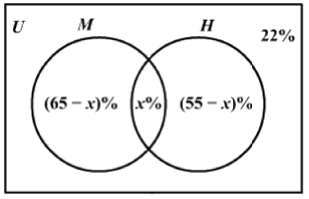65 - x + x + 55 - x = 100 - 22

x = 42%
Now, 42% i.e. 48909 students passed in both the subjects.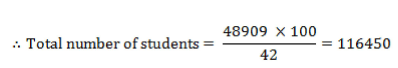Answer: 116450

QUESTION: 23

x is a positive integer with value at most equal to 110. How many values of x are possible if x is not a factor of (x - 1)!?

Solution:

If x is a prime number greater than 1, then x is not a factor of (x - 1)!.
There are 29 primes upto 110. 4 is also one such number.
Hence, option 4.

QUESTION: 24

A person starts travelling from a point P towards R passes through Q which is between P and R. The time taken to travel from P to Q is half that of the time taken from Q to R. However, after passing through any of the given points, he travels at a speed which is two-thirds that of the previous journey. Find the time taken, in seconds, to travel from P to R and back to P, if he always passes through Q in the journey and takes 15 minutes to go from P to Q initially.

Solution:

Let the initial speed of the person be x and the time taken to go from P to Q be t.
The distance from Q to R = 4xt / 3

The time taken to go from R to Q = (4xt / 3) / (4x / 9) = 3t

The time taken to go from Q to P = xt / (8x / 27) = (27 / 8)t

Hence, the total time taken = t + 2t + 3t + (27 / 8)t = (75 / 8)t = 9.375t

Now, t = 15 minutes = 900 seconds

the total time taken = 8437.5 seconds.
Hence, option 4.

QUESTION: 25

x2 - 3x + 2 > 0, y2 - 4y + 3 > 0. If x, y are positive integers and xy = 16, then find the value of Ix-yI.

Solution:

x2 - 3x + 2 > 0 is true when x < 1, x > 2 ... (i) y2 - 4y + 3 > 0 is true wheny < 1, y > 3 ... (ii)
Now,

xy = 16
The possible values of (x, y) are (1, 16), (2, 8), (4, 4), (8, 2) and (16, 1).
From (i) and (ii), we can see that only (4,4) satisifies both the conditions. |x - y | = |4 - 4 | = 0

Hence, option 2.

QUESTION: 26

Find the minimum value of the quadratic expression 3x2 + 11x - 23.

Solution:

The minimum value of a quadratic expression ax2 + bx + c (when a > 0)

occurs at x = -b / 2a and its minimum value is -(b2 - 4ac) / 4a

The minimum value of the equation is [4(3)(-23) - (11)2] / 12 = -397 / 12

Hence, option 4.

QUESTION: 27

50 square stone slabs of equal size were needed to cover, a floor area of 72 sq. m. The length of each stone slab is :

Solution:
QUESTION: 28

A and B start working on a project. After 10 days B is replaced by C who can complete the project alone in 20 days. If A and B together can complete the project in 30 days, then find the day on which the project will be completed after C replaces B, if the efficiency of B is twice that of A.

Solution:

Let the number of days required by B alone to complete the project be x.
The number of days required by A alone to complete it = 2x

1/x + l/2x = 1/30

x = 45
The part of work done in 10 days =1/3

Work donee by A and C in 1 day = (1/90) + (1/20) = (11/180)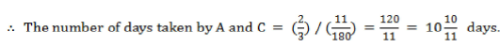Hence, option 2.

QUESTION: 29

37% of the total number of people in a city read newspaper ABC and exceed the number of people who read XYZ by 8000. If 27% of the total population do not read any newspaper then find the population of the city if there are only two newspapers in the city. [Assume that no one reads more than one newspaper].

Solution:

Let the total population of the town be x.
The number of people who read XYZ = 0.37x - 8000

0.37x+0.37x - 8000 + 0.27x = x

x = 8,00,000

Hence, option 1.

QUESTION: 30

If x2 + x - 2 < 0; then which of the following statement is true?

1. x - 1 > 0

2. x - 1 < 0

3. x + 2 > 0

4. x - 2 < 0

Solution:

x2 + x - 2 < 0

(x - 1) (x + 2) < 0

(x - 1) < 0 and (x + 2) > 0 or (x - 1) > 0 and (x + 2) < 0

x < 1, x > -2 or x > 1, x < -2 which is not possible.

-2 < x < 1

(x - 1) < 0 and (x + 2) > 0

Hence, option 3.

QUESTION: 31

At 12:00 AM both the hour and a minute hand were overlapping each other. How many more minutes does the minute hand travel than the hour hand in the next 54 minutes?

Solution:

When minute hand travels 60 minutes, hour hand travels 5 minutes.
The minute hand travels 55 minutes more than the hour hand in an hour.

In 54 minutes, the Minute hand travels = (54 x 55/ 60) = 49.5

Hence, option 3.

QUESTION: 32

A and B start working together on a project and both have the same efficiency in the beginning. However the efficiency of A decreases to 0.6 times the usual after working for 2 hours. If the project can be finished in 120 man hours, then find the minimum number of days required to finish the work if both do an equal manhours of work each day and the sum of the total number of hours of work by both each day is 12. [ Initial efficiency of A = Efficiency of B = 1 man hour].

Solution:

Let the number of hours worked by B be x.
The hours worked by A = (12 - x)

The manhours of work finished by A = (12 - x - 2) x 0.6 + 2

(12 - x - 2) x 0.6 + 2 = x

x = 5
The total manhours o f work done by them in a day = 2*x = 2 x 5 = 10

Thus, the number of days required to finsih the work = 120/10 =12 days

Hence, option 2.

QUESTION: 33

The average number of apples per carton for three cartons of apple is 90. The average price per carton is Rs.1500. The average price of apples in the two cartons which do not contain the highest number of apples is 20 and 25 respectively. The price of the carton with the highest number of apples is 1900. If the highest difference in the number of apples in any two cartons is 100, then find the lowest number of apples in a carton.

Solution:

Let the number of apples in the carton with least number of apples be x and second least number of apples be y.

x +y + x + 100 = 270

2x +y = 170...(i)

Also, the total amount spent on the two cartons with x and y apples = 4500 - 1900 = 2600
20x + 25y = 2600

4x + 5y = 520.. .(ii)

From (i) and (ii)

x = 55,y = 60

The lowest number of number of apples in a cartoon = 55.
Hence, option 3.

*Answer can only contain numeric values
QUESTION: 34

A saree is one-fourth by length green, two-fifth by length red and the remaining 3850 cm by length is black. What is the length of the saree in meters?

Solution:

Let the length of the saree be ‘x’ cm.

x - (1 / 4)x - (2 / 5)x = 3850

(7 / 20)x = 3850

x = 11000 cm = 110 m